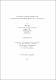## Fractional Brownian motion and managing risk with short-term futures contracts2016
##### Publisher
University of Alabama Libraries
##### Abstract

In this dissertation, we consolidate previous research done by , , ,  and  on an optimal strategy to reduce the running risk in hedging a long-term supply commitment with short-term futures contracts. Under the assumption that the market price of the commodity is modeled by the fractional Brownian motion (fBm), we study the following optimization problem: Under the constraint $$\ \int_{0}^{1}\int_{0}^{1}(1-g(u))(1-g(v))|u-v|^{2H-2}dudv\leq\theta,$$ Which measurable function (g: [0,1]\rightarrow R[0,1]) will minimize the value of $$\ \sup_{t\in[0,1]}\int_{0}^{t}\int_{0}^{t}(t-g(u))(t-g(v))|u-v|^{2H-2}dudv?$$ where (\theta\in[0,\frac{H^{2H}}{(H+1)^{2H+2}}]) and (H\in(\frac{1}{2},1)). Under the fractional market model, we gave the spot risk function by using the hedging strategies provided by Glasserman  and found that when the hurst index (H) is equal to (0.5) the maximal spot risk is the same as the result given in . The main work in this dissertation is to show that a unique solution to this optimization problem always exists.

##### Description
Electronic Thesis or Dissertation
Mathematics